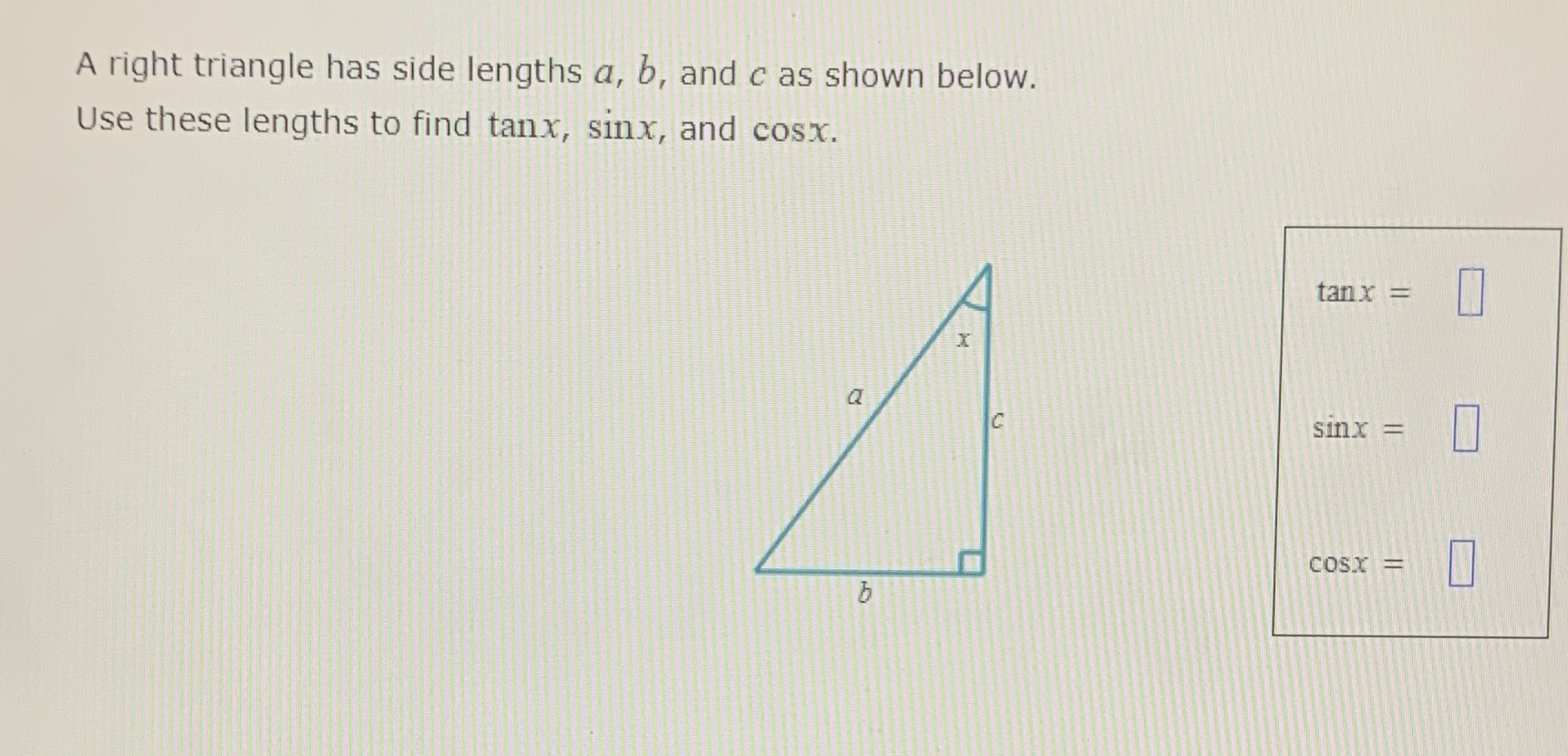### Still have math questions?A right triangle has side lengths $$a , b ,$$ and $$c$$ as shown below. Use these lengths to find $$\tan x , \sin x ,$$ and $$\cos x$$ .
$$sinx = \frac{b}{a } \\\space \\cosx = \frac{c}{a } \\\space \\tanx = \frac{b}{c }$$